# fft

Fast Fourier transform

## Syntax

``Y = fft(X)``
``Y = fft(X,n)``
``Y = fft(X,n,dim)``

## Description

example

````Y = fft(X)` computes the discrete Fourier transform (DFT) of `X` using a fast Fourier transform (FFT) algorithm. If `X` is a vector, then `fft(X)` returns the Fourier transform of the vector.If `X` is a matrix, then `fft(X)` treats the columns of `X` as vectors and returns the Fourier transform of each column.If `X` is a multidimensional array, then `fft(X)` treats the values along the first array dimension whose size does not equal 1 as vectors and returns the Fourier transform of each vector. ```

example

````Y = fft(X,n)` returns the `n`-point DFT. If no value is specified, `Y` is the same size as `X`. If `X` is a vector and the length of `X` is less than `n`, then `X` is padded with trailing zeros to length `n`.If `X` is a vector and the length of `X` is greater than `n`, then `X` is truncated to length `n`.If `X` is a matrix, then each column is treated as in the vector case.If `X` is a multidimensional array, then the first array dimension whose size does not equal 1 is treated as in the vector case. ```

example

````Y = fft(X,n,dim)` returns the Fourier transform along the dimension `dim`. For example, if `X` is a matrix, then `fft(X,n,2)` returns the n-point Fourier transform of each row.```

## Examples

collapse all

Use Fourier transforms to find the frequency components of a signal buried in noise.

Specify the parameters of a signal with a sampling frequency of 1 kHz and a signal duration of 1.5 seconds.

```Fs = 1000; % Sampling frequency T = 1/Fs; % Sampling period L = 1500; % Length of signal t = (0:L-1)*T; % Time vector```

Form a signal containing a 50 Hz sinusoid of amplitude 0.7 and a 120 Hz sinusoid of amplitude 1.

`S = 0.7*sin(2*pi*50*t) + sin(2*pi*120*t);`

Corrupt the signal with zero-mean white noise with a variance of 4.

`X = S + 2*randn(size(t));`

Plot the noisy signal in the time domain. It is difficult to identify the frequency components by looking at the signal `X(t)`.

```plot(1000*t(1:50),X(1:50)) title('Signal Corrupted with Zero-Mean Random Noise') xlabel('t (milliseconds)') ylabel('X(t)')```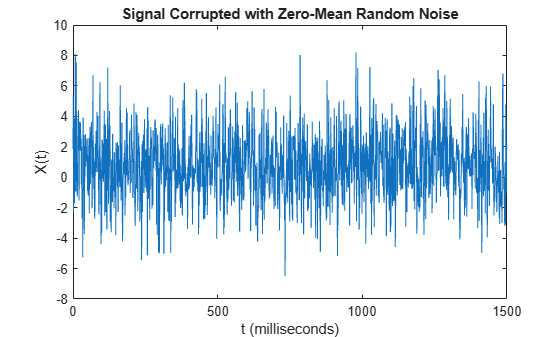Compute the Fourier transform of the signal.

`Y = fft(X);`

Compute the two-sided spectrum `P2`. Then compute the single-sided spectrum `P1` based on `P2` and the even-valued signal length `L`.

```P2 = abs(Y/L); P1 = P2(1:L/2+1); P1(2:end-1) = 2*P1(2:end-1);```

Define the frequency domain `f` and plot the single-sided amplitude spectrum `P1`. The amplitudes are not exactly at 0.7 and 1, as expected, because of the added noise. On average, longer signals produce better frequency approximations.

```f = Fs*(0:(L/2))/L; plot(f,P1) title('Single-Sided Amplitude Spectrum of X(t)') xlabel('f (Hz)') ylabel('|P1(f)|')```Now, take the Fourier transform of the original, uncorrupted signal and retrieve the exact amplitudes, 0.7 and 1.0.

```Y = fft(S); P2 = abs(Y/L); P1 = P2(1:L/2+1); P1(2:end-1) = 2*P1(2:end-1); plot(f,P1) title('Single-Sided Amplitude Spectrum of S(t)') xlabel('f (Hz)') ylabel('|P1(f)|')```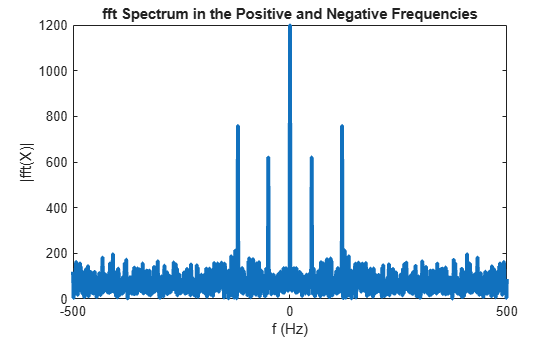Convert a Gaussian pulse from the time domain to the frequency domain.

Define signal parameters and a Gaussian pulse, `X`.

```Fs = 100; % Sampling frequency t = -0.5:1/Fs:0.5; % Time vector L = length(t); % Signal length X = 1/(4*sqrt(2*pi*0.01))*(exp(-t.^2/(2*0.01)));```

Plot the pulse in the time domain.

```plot(t,X) title('Gaussian Pulse in Time Domain') xlabel('Time (t)') ylabel('X(t)')```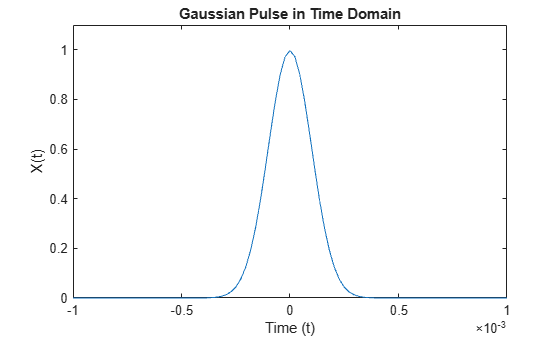To use the `fft` function to convert the signal to the frequency domain, first identify a new input length that is the next power of 2 from the original signal length. This will pad the signal `X` with trailing zeros in order to improve the performance of `fft`.

`n = 2^nextpow2(L);`

Convert the Gaussian pulse to the frequency domain.

`Y = fft(X,n);`

Define the frequency domain and plot the unique frequencies.

```f = Fs*(0:(n/2))/n; P = abs(Y/n).^2; plot(f,P(1:n/2+1)) title('Gaussian Pulse in Frequency Domain') xlabel('Frequency (f)') ylabel('|P(f)|^2')```Compare cosine waves in the time domain and the frequency domain.

Specify the parameters of a signal with a sampling frequency of 1kHz and a signal duration of 1 second.

```Fs = 1000; % Sampling frequency T = 1/Fs; % Sampling period L = 1000; % Length of signal t = (0:L-1)*T; % Time vector```

Create a matrix where each row represents a cosine wave with scaled frequency. The result, `X`, is a 3-by-1000 matrix. The first row has a wave frequency of 50, the second row has a wave frequency of 150, and the third row has a wave frequency of 300.

```x1 = cos(2*pi*50*t); % First row wave x2 = cos(2*pi*150*t); % Second row wave x3 = cos(2*pi*300*t); % Third row wave X = [x1; x2; x3];```

Plot the first 100 entries from each row of `X` in a single figure in order and compare their frequencies.

```for i = 1:3 subplot(3,1,i) plot(t(1:100),X(i,1:100)) title(['Row ',num2str(i),' in the Time Domain']) end```For algorithm performance purposes, `fft` allows you to pad the input with trailing zeros. In this case, pad each row of `X` with zeros so that the length of each row is the next higher power of 2 from the current length. Define the new length using the `nextpow2` function.

`n = 2^nextpow2(L);`

Specify the `dim` argument to use `fft` along the rows of `X`, that is, for each signal.

`dim = 2;`

Compute the Fourier transform of the signals.

`Y = fft(X,n,dim);`

Calculate the double-sided spectrum and single-sided spectrum of each signal.

```P2 = abs(Y/L); P1 = P2(:,1:n/2+1); P1(:,2:end-1) = 2*P1(:,2:end-1);```

In the frequency domain, plot the single-sided amplitude spectrum for each row in a single figure.

```for i=1:3 subplot(3,1,i) plot(0:(Fs/n):(Fs/2-Fs/n),P1(i,1:n/2)) title(['Row ',num2str(i),' in the Frequency Domain']) end```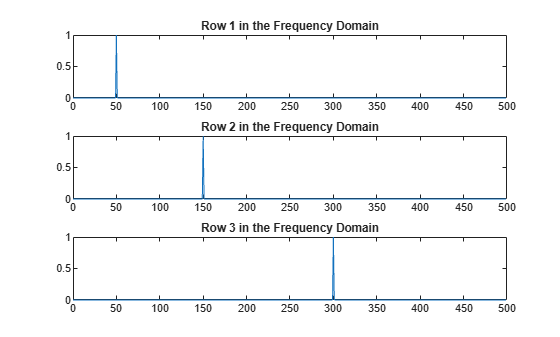## Input Arguments

collapse all

Input array, specified as a vector, matrix, or multidimensional array.

If `X` is an empty 0-by-0 matrix, then `fft(X)` returns an empty 0-by-0 matrix.

Data Types: `double` | `single` | `int8` | `int16` | `int32` | `uint8` | `uint16` | `uint32` | `logical`
Complex Number Support: Yes

Transform length, specified as `[]` or a nonnegative integer scalar. Specifying a positive integer scalar for the transform length can increase the performance of `fft`. The length is typically specified as a power of 2 or a value that can be factored into a product of small prime numbers. If `n` is less than the length of the signal, then `fft` ignores the remaining signal values past the `n`th entry and returns the truncated result. If `n` is `0`, then `fft` returns an empty matrix.

Example: `n = 2^nextpow2(size(X,1))`

Data Types: `double` | `single` | `int8` | `int16` | `int32` | `uint8` | `uint16` | `uint32` | `logical`

Dimension to operate along, specified as a positive integer scalar. If you do not specify the dimension, then the default is the first array dimension of size greater than 1.

• `fft(X,[],1)` operates along the columns of `X` and returns the Fourier transform of each column.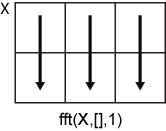• `fft(X,[],2)` operates along the rows of `X` and returns the Fourier transform of each row.If `dim` is greater than `ndims(X)`, then `fft(X,[],dim)` returns `X`. When `n` is specified, `fft(X,n,dim)` pads or truncates `X` to length `n` along dimension `dim`.

Data Types: `double` | `single` | `int8` | `int16` | `int32` | `uint8` | `uint16` | `uint32` | `logical`

## Output Arguments

collapse all

Frequency domain representation returned as a vector, matrix, or multidimensional array.

If `X` is of type `single`, then `fft` natively computes in single precision, and `Y` is also of type `single`. Otherwise, `Y` is returned as type `double`.

The size of `Y` is as follows:

• For `Y = fft(X)` or ```Y = fft(X,[],dim)```, the size of `Y` is equal to the size of `X`.

• For `Y = fft(X,n,dim)`, the value of `size(Y,dim)` is equal to `n`, while the size of all other dimensions remains as in `X`.

If `X` is real, then `Y` is conjugate symmetric, and the number of unique points in `Y` is `ceil((n+1)/2)`.

Data Types: `double` | `single`

collapse all

### Discrete Fourier Transform of Vector

`Y = fft(X)` and ```X = ifft(Y)``` implement the Fourier transform and inverse Fourier transform, respectively. For `X` and `Y` of length `n`, these transforms are defined as follows:

`$\begin{array}{l}Y\left(k\right)=\sum _{j=1}^{n}X\left(j\right)\text{\hspace{0.17em}}{W}_{n}^{\left(j-1\right)\text{​}\left(k-1\right)}\\ X\left(j\right)=\frac{1}{n}\sum _{k=1}^{n}Y\left(k\right)\text{\hspace{0.17em}}{W}_{n}{}^{-\left(j-1\right)\text{​}\left(k-1\right)},\end{array}$`

where

`${W}_{n}={e}^{\left(-2\pi i\right)/n}$`

is one of n roots of unity.

## Tips

• The execution time for `fft` depends on the length of the transform. Transform lengths that have only small prime factors are significantly faster than those that are prime or have large prime factors.

• For most values of `n`, real-input DFTs require roughly half the computation time of complex-input DFTs. However, when `n` has large prime factors, there is little or no speed difference.

• You can potentially increase the speed of `fft` using the utility function, `fftw`. This function controls the optimization of the algorithm used to compute an FFT of a particular size and dimension.

## Algorithms

The FFT functions (`fft`, `fft2`, `fftn`, `ifft`, `ifft2`, `ifftn`) are based on a library called FFTW  .

 Frigo, M., and S. G. Johnson. “FFTW: An Adaptive Software Architecture for the FFT.” Proceedings of the International Conference on Acoustics, Speech, and Signal Processing. Vol. 3, 1998, pp. 1381-1384.